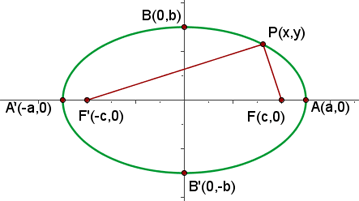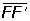Learn from home

The teachersThe ellipse is the locus of points on the plane whose sum of distances to two fixed points, foci, are always constant.## Elements of the Ellipse

Foci

The foci are the fixed points of the ellipse which are located on the major axis. They are denoted by F and F'.

Major Axis

The major axis of the ellipse is the line segment, which has a length of 2a.

The major axis coincides with the major diameter and passes through the center point and both foci.

Minor Axis

The minor axis of the ellipse is the line segment, which has a length of 2b.

The minor axis is the perpendicular bisector of the major axis.

Focal Length

The focal length of the ellipse is the line segment, which has a length of 2c.

Center

The center of the ellipse is the point of intersection of the axes. It is the center of symmetry of the ellipse.

Vertices

The vertices of the ellipse are the points of intersection of the ellipse with the axes. They are denoted by A, A', B and B'.

The focal radii are the line segments that join a point on the ellipse with both foci. They are denoted by PF and PF'.

Semi-Major Axis

The semi-major axis is the line segment that runs from the centre of the ellipse, through a focus, and to a vertex of the ellipse. Its length is a.

Semi-Minor Axis

The semi-minor axis is the line segment, perpendicular to the semi-major axis, that runs from the centre of the ellipse to a vertex. Its length is b.

If a = b, an ellipse is more accurately defined as a circle.

Axes of Symmetry

The axes of symmetry are the lines that coincide with the major and minor axes.

### Relationship between the SemiaxesDid you like the article?(2 votes, average: 5.00 out of 5)Loading...

Emma

I am passionate about travelling and currently live and work in Paris. I like to spend my time reading, gardening, running, learning languages and exploring new places.

Did you like
this resource?

Bravo!